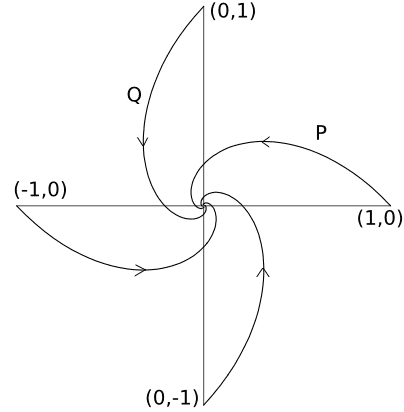# Four Bugs Differential Equation

simpleman008

## Homework Statement

Four bugs are walking on a flat surface. They start at the four points (1,1), (1,-1), (-1,1), and (-1,-1) and begin walking counterclockwise, each one following the next. Show that the motion of bug A, who starts at (1,1), satisfies the equation $$\frac{dy}{dx}$$= $$\frac{y-x}{x-y}$$ and solve using an appropriate substitution.

## Homework Equations

After some extensive research, I've found the same problem on another website (http://legacy.lclark.edu/~istavrov/diffeq-revsheet1-09.pdf)
that states the motion of the bug starting from (1,-1) satisfies $$\frac{dy}{dx}$$= $$\frac{y-x}{x+y}$$ and that each bugs motion can be shown by the graph:.

## The Attempt at a Solution

A hint from the website, as well as from my teacher, was given saying that y=ux would be the most viable substitution. So starting there I have
$$y=ux => dy=xdu + udx$$
$$\frac{xdu + udx}{dx}$$ = $$\frac{ux-x}{x-ux}$$

which after some algebra leads me to:
$$(x-xu+u)du$$= $$(u^{2}+u-1)dx$$
This is where I'm stuck, and it doesn't even help me with the first part about proving WHY dy/dx is what it is (i have ideas floating around in my head but nothings clicking yet or coming together in any meaningful way). Any and all help is greatly appreciated!

Last edited by a moderator:

## Answers and Replies

Staff Emeritus
Gold Member
2021 Award
Are you sure the equation for the first part is right? Because $$\frac{y-x}{x-y}=-1$$ which doesn't make for a very interesting differential equation

simpleman008
Are you sure the equation for the first part is right? Because $$\frac{y-x}{x-y}=-1$$ which doesn't make for a very interesting differential equation

Yes that is how he had it written, but why did you put it equal to -1?

simpleman008
Also let it be noted that the graph I included obviously isn't correct cause it needs to be rotated 45 degrees